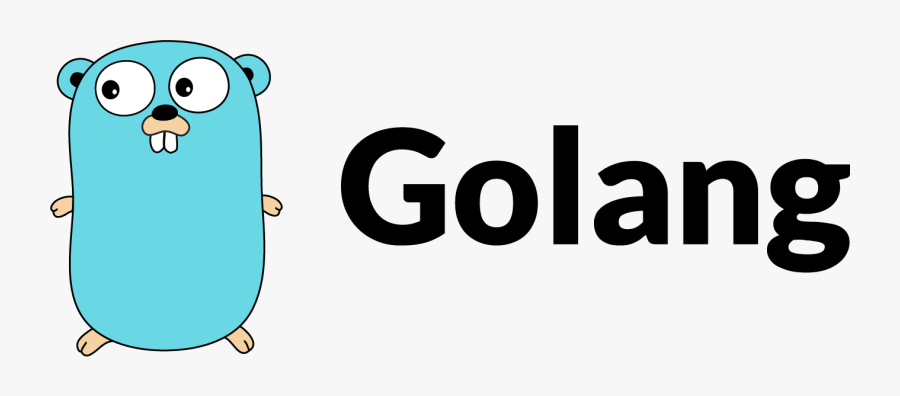# Generating `Equal` methods for Go structs with `goderive`Sometimes you need to check the equality of two `struct`s in Go, and depending on how complicated they are, you probably want to avoid hand-writing the `Equal` method if possible, and you want to avoid `reflect.DeepEqual` for performance reasons, at least in your production code.

If I were writing Java, I'd ask IntelliJ to generate an `equals` method for me, but wanted to find out if Go had a tool for this, because there usually is one. I asked around in the Go communities I'm part of, and my colleague Michal suggested looking at `goderive`, which worked out very nicely for what I needed.

Although `goderive` supports many methods being generated, I only needed an `Equal` method.

Let's say that we have a slightly complex struct setup which results in this set of types:

``````type ComplexStruct struct {
Int      int
MyStruct MyStruct
Anon     Anon
}

type Anon struct {
Nested Nested
}

type Nested struct {
MyStruct MyStruct
}

type MyStruct struct {
Int64     int64
StringPtr *string
}
``````

To create our own `Equal` method is OK for us to do, but we can instead use `goderive` to do the heavy lifting, by creating:

``````func (this *ComplexStruct) Equal(that *ComplexStruct) bool {
return deriveEqualComplexStruct(this, that)
}
``````

This will fail to compile - but that's OK, running `goderive` will then auto-generate the file `derived.gen.go` which contains the implementation we need:

``````// deriveEqualComplexStruct returns whether this and that are equal.
func deriveEqualComplexStruct(this, that *ComplexStruct) bool {
return (this == nil && that == nil) ||
this != nil && that != nil &&
this.Int == that.Int &&
deriveEqual(&this.MyStruct, &that.MyStruct) &&
deriveEqual_(&this.Anon, &that.Anon)
}

// deriveEqual returns whether this and that are equal.
func deriveEqual(this, that *MyStruct) bool {
return (this == nil && that == nil) ||
this != nil && that != nil &&
this.Int64 == that.Int64 &&
((this.StringPtr == nil && that.StringPtr == nil) || (this.StringPtr != nil && that.StringPtr != nil && *(this.StringPtr) == *(that.StringPtr)))
}

// deriveEqual_ returns whether this and that are equal.
func deriveEqual_(this, that *Anon) bool {
return (this == nil && that == nil) ||
this != nil && that != nil &&
deriveEqual_1(&this.Nested, &that.Nested)
}

// deriveEqual_1 returns whether this and that are equal.
func deriveEqual_1(this, that *Nested) bool {
return (this == nil && that == nil) ||
this != nil && that != nil &&
deriveEqual(&this.MyStruct, &that.MyStruct)
}
``````

This then generates a set of methods which check the equality for all the types involved - awesome 👏

A sample project for this can be found on GitLab.com.

Written byJamie Tanna on , and last updated on .